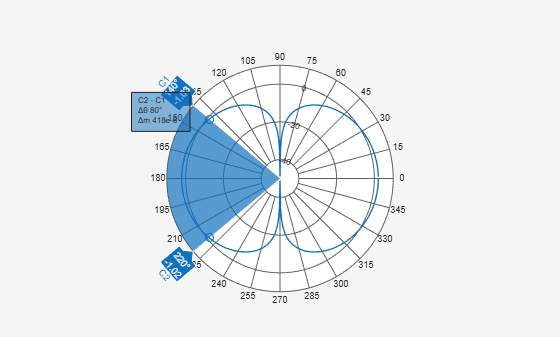# beamwidth

Beamwidth of antenna

## Syntax

``beamwidth(antenna,frequency,azimuth,elevation)``
``bw = beamwidth(antenna,frequency,azimuth,elevation,dBdown)``
``````[bw,angles] = beamwidth(____)``````

## Description

example

````beamwidth(antenna,frequency,azimuth,elevation)` plots the beamwidth of the input antenna at a specified frequency. The beamwidth is the angular separation at which the magnitude of the directivity pattern decreases by a certain value from the peak of the main beam. The directivity decreases in the direction specified by azimuth and elevation angles of the antenna. Note`beamwidth` plots only one beamwidth for symmetrical patterns.`beamwidth` might not interpret the data well for partial angle data. ```
````bw = beamwidth(antenna,frequency,azimuth,elevation,dBdown)` returns the beamwidth of the antenna at a specified `dBdown` value from the peak of the radiation pattern main beam.```

example

``````[bw,angles] = beamwidth(____)``` returns the beamwidth and angles (points in a plane) using any input arguments from previous syntaxes.```

## Examples

collapse all

Plot the beamwidth for a dipole antenna at azimuth=0 and elevation=1:1:360 (x-z plane)

```d = dipole; beamwidth(d,70e6,0,1:1:360);```Calculate the beamwidth of a helix antenna and the angles of the beamwidth. The antenna has an azimuth angle of 1:1:360 degrees, an elevation angle of 0 degrees on the X-Y plane, and a dB down value of 5 dB.

```hx = helix; [bw,angles] = beamwidth(hx,2e9,1:1:360,0,5)```
```bw = 145 ```
```angles = 1×2 143 288 ```

Create a `fractalGasket `antenna object`.`

`fg = fractalGasket("NumIterations",4,"TiltAxis",[0 1 0],'Tilt',90);`

Calculate beamwidth and angle of a `fractalGasket.`

`[bw,ang] = beamwidth(fg,1.3e9,0,0:1:360) % bw is a 2-by-1 vector.`
```bw = 2×1 24.0000 24.0000 ```
```ang = 2×2 348 12 168 192 ```

Plot beamwidth.

`beamwidth(fg,1.3e9,0,0:1:360)`Plot Second Beamwidth Solution

Get the `polarpattern `handle.

`P = polarpattern('gco'); `

Hide the beamwidth span and remove the cursor C1 and C2. All the cursors can also be removed using the function `removeCursors.`

```showSpan(P,0); removeCursors(P,1); removeCursors(P,2); ```

Add the cursors at other side of the plot and second beamwidth solution is displayed.

```addCursor(P,ang(2,:)); showSpan(P,1); ```## Input Arguments

collapse all

Antenna object, specified as a scalar handle.

Frequency to calculate beamwidth, specified as a scalar in Hz.

Example: 70e6

Data Types: `double`

Azimuth angle of the antenna, specified as a scalar or vector in degrees. If the elevation angle is specified as a vector, then the azimuth angle must be a scalar.

Example: 3

Data Types: `double`

Elevation angle of the antenna, specified as a scalar or vector in degrees. If the azimuth angle is specified as a vector, then the elevation angle must be a scalar.

Example: 1:1:360

Data Types: `double`

Power point from peak of main beam of antenna, specified as a scalar in dB.

Example: 5

Data Types: `double`

## Output Arguments

collapse all

Beamwidth of antenna, returned as a scalar in degrees or a 2-by-1 vector with each element unit in degrees.

Points on plane used to measure beamwidth, returned as a vector with each element unit in degrees.